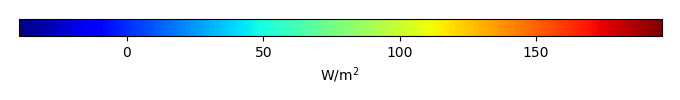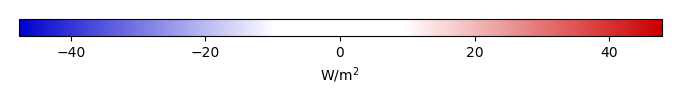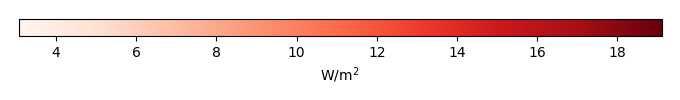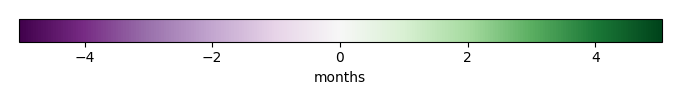# Mean State

Period Mean (original grids) [W/m2]
Model Period Mean (intersection) [W/m2]
Model Period Mean (complement) [W/m2]
Benchmark Period Mean (intersection) [W/m2]
Benchmark Period Mean (complement) [W/m2]
Bias [W/m2]
RMSE [W/m2]
Phase Shift [months]
Bias Score 
RMSE Score 
Seasonal Cycle Score 
Spatial Distribution Score 
Interannual Variability Score 
Overall Score 
Benchmark [-] 131.
CLM4 [-] 120. 121. 130. 157. -8.85 20.7 1.31 0.603 0.420 0.827 0.982 0.716 0.661
CLM4.5 [-] 117. 117. 130. 157. -12.6 22.4 1.29 0.535 0.421 0.829 0.996 0.719 0.653
CLM5 [-] 116. 116. 130. 157. -13.7 22.6 1.40 0.513 0.420 0.812 0.990 0.694 0.642
Period Mean (original grids) [W/m2]
Model Period Mean (intersection) [W/m2]
Model Period Mean (complement) [W/m2]
Benchmark Period Mean (intersection) [W/m2]
Benchmark Period Mean (complement) [W/m2]
Bias [W/m2]
RMSE [W/m2]
Phase Shift [months]
Bias Score 
RMSE Score 
Seasonal Cycle Score 
Spatial Distribution Score 
Interannual Variability Score 
Overall Score 
Benchmark [-] 123.
CLM4 [-] 103. 102. 122. 160. -19.6 26.7 1.30 0.469 0.489 0.816 0.996 0.713 0.662
CLM4.5 [-] 98.3 97.8 122. 160. -23.9 29.8 1.38 0.390 0.497 0.807 0.916 0.708 0.636
CLM5 [-] 97.4 96.9 122. 160. -24.8 30.3 1.43 0.374 0.505 0.789 0.941 0.650 0.627
Period Mean (original grids) [W/m2]
Model Period Mean (intersection) [W/m2]
Model Period Mean (complement) [W/m2]
Benchmark Period Mean (intersection) [W/m2]
Benchmark Period Mean (complement) [W/m2]
Bias [W/m2]
RMSE [W/m2]
Phase Shift [months]
Bias Score 
RMSE Score 
Seasonal Cycle Score 
Spatial Distribution Score 
Interannual Variability Score 
Overall Score 
Benchmark [-] 123.
CLM4 [-] 110. 110. 121. 157. -10.8 22.7 1.20 0.688 0.584 0.832 0.983 0.737 0.735
CLM4.5 [-] 104. 104. 121. 157. -15.9 25.0 1.16 0.594 0.586 0.836 0.941 0.747 0.715
CLM5 [-] 105. 105. 121. 157. -15.0 25.1 1.49 0.616 0.570 0.774 0.955 0.683 0.695
Period Mean (original grids) [W/m2]
Model Period Mean (intersection) [W/m2]
Model Period Mean (complement) [W/m2]
Benchmark Period Mean (intersection) [W/m2]
Benchmark Period Mean (complement) [W/m2]
Bias [W/m2]
RMSE [W/m2]
Phase Shift [months]
Bias Score 
RMSE Score 
Seasonal Cycle Score 
Spatial Distribution Score 
Interannual Variability Score 
Overall Score 
Benchmark [-] 34.4
CLM4 [-] 28.6 28.7 34.5 33.0 -6.52 21.2 0.303 0.848 0.722 0.980 0.907 0.728 0.818
CLM4.5 [-] 33.5 33.6 34.5 33.0 -2.29 20.4 0.251 0.864 0.729 0.983 0.833 0.709 0.808
CLM5 [-] 32.9 33.0 34.5 33.0 -3.11 20.8 0.266 0.864 0.725 0.982 0.819 0.736 0.808
Period Mean (original grids) [W/m2]
Model Period Mean (intersection) [W/m2]
Model Period Mean (complement) [W/m2]
Benchmark Period Mean (intersection) [W/m2]
Benchmark Period Mean (complement) [W/m2]
Bias [W/m2]
RMSE [W/m2]
Phase Shift [months]
Bias Score 
RMSE Score 
Seasonal Cycle Score 
Spatial Distribution Score 
Interannual Variability Score 
Overall Score 
Benchmark [-] 147.
CLM4 [-] 135. 135. 145. 156. -6.56 19.0 1.70 0.590 0.425 0.729 0.997 0.715 0.647
CLM4.5 [-] 132. 132. 145. 156. -8.14 19.6 1.72 0.563 0.427 0.726 0.994 0.709 0.641
CLM5 [-] 128. 127. 145. 156. -13.0 21.7 1.63 0.478 0.433 0.743 0.986 0.685 0.626
Period Mean (original grids) [W/m2]
Model Period Mean (intersection) [W/m2]
Model Period Mean (complement) [W/m2]
Benchmark Period Mean (intersection) [W/m2]
Benchmark Period Mean (complement) [W/m2]
Bias [W/m2]
RMSE [W/m2]
Phase Shift [months]
Bias Score 
RMSE Score 
Seasonal Cycle Score 
Spatial Distribution Score 
Interannual Variability Score 
Overall Score 
Benchmark [-] 53.7
CLM4 [-] 53.1 53.1 53.5 64.9 -0.194 15.4 0.213 0.890 0.795 0.986 0.807 0.672 0.824
CLM4.5 [-] 50.6 50.6 53.5 64.9 -2.66 16.7 0.211 0.877 0.781 0.986 0.824 0.684 0.822
CLM5 [-] 48.1 48.1 53.5 64.9 -5.14 17.0 0.214 0.875 0.774 0.986 0.903 0.721 0.839
Period Mean (original grids) [W/m2]
Model Period Mean (intersection) [W/m2]
Model Period Mean (complement) [W/m2]
Benchmark Period Mean (intersection) [W/m2]
Benchmark Period Mean (complement) [W/m2]
Bias [W/m2]
RMSE [W/m2]
Phase Shift [months]
Bias Score 
RMSE Score 
Seasonal Cycle Score 
Spatial Distribution Score 
Interannual Variability Score 
Overall Score 
Benchmark [-] 71.7
CLM4 [-] 65.3 65.3 71.1 90.6 -4.60 18.6 0.465 0.828 0.719 0.957 0.984 0.692 0.816
CLM4.5 [-] 64.0 63.9 71.1 90.6 -5.79 18.6 0.453 0.821 0.720 0.959 0.989 0.688 0.816
CLM5 [-] 62.6 62.6 71.1 90.6 -7.26 19.0 0.429 0.810 0.717 0.964 0.994 0.706 0.818
Period Mean (original grids) [W/m2]
Model Period Mean (intersection) [W/m2]
Model Period Mean (complement) [W/m2]
Benchmark Period Mean (intersection) [W/m2]
Benchmark Period Mean (complement) [W/m2]
Bias [W/m2]
RMSE [W/m2]
Phase Shift [months]
Bias Score 
RMSE Score 
Seasonal Cycle Score 
Spatial Distribution Score 
Interannual Variability Score 
Overall Score 
Benchmark [-] 120.
CLM4 [-] 100. 99.8 118. 153. -13.9 24.9 0.621 0.626 0.543 0.930 0.978 0.727 0.724
CLM4.5 [-] 96.2 95.8 118. 153. -17.4 26.6 0.647 0.562 0.549 0.927 0.983 0.707 0.713
CLM5 [-] 95.0 94.6 118. 153. -18.8 27.5 0.690 0.545 0.541 0.922 0.994 0.647 0.698
Period Mean (original grids) [W/m2]
Model Period Mean (intersection) [W/m2]
Model Period Mean (complement) [W/m2]
Benchmark Period Mean (intersection) [W/m2]
Benchmark Period Mean (complement) [W/m2]
Bias [W/m2]
RMSE [W/m2]
Phase Shift [months]
Bias Score 
RMSE Score 
Seasonal Cycle Score 
Spatial Distribution Score 
Interannual Variability Score 
Overall Score 
Benchmark [-] 30.5
CLM4 [-] 20.8 21.2 31.0 19.5 -10.5 21.1 0.246 0.822 0.724 0.984 0.981 0.706 0.823
CLM4.5 [-] 23.9 24.2 31.0 19.5 -7.74 19.6 0.249 0.855 0.733 0.983 0.981 0.709 0.832
CLM5 [-] 24.2 24.5 31.0 19.5 -7.63 19.3 0.205 0.860 0.736 0.986 0.981 0.754 0.842
Period Mean (original grids) [W/m2]
Model Period Mean (intersection) [W/m2]
Model Period Mean (complement) [W/m2]
Benchmark Period Mean (intersection) [W/m2]
Benchmark Period Mean (complement) [W/m2]
Bias [W/m2]
RMSE [W/m2]
Phase Shift [months]
Bias Score 
RMSE Score 
Seasonal Cycle Score 
Spatial Distribution Score 
Interannual Variability Score 
Overall Score 
Benchmark [-] 109.
CLM4 [-] 94.8 94.7 108. 131. -11.2 19.5 0.495 0.760 0.731 0.963 0.951 0.780 0.819
CLM4.5 [-] 94.1 94.1 108. 131. -11.7 20.0 0.518 0.748 0.728 0.960 0.940 0.788 0.816
CLM5 [-] 93.6 93.6 108. 131. -12.2 20.1 0.514 0.751 0.727 0.960 0.956 0.769 0.815
Period Mean (original grids) [W/m2]
Model Period Mean (intersection) [W/m2]
Model Period Mean (complement) [W/m2]
Benchmark Period Mean (intersection) [W/m2]
Benchmark Period Mean (complement) [W/m2]
Bias [W/m2]
RMSE [W/m2]
Phase Shift [months]
Bias Score 
RMSE Score 
Seasonal Cycle Score 
Spatial Distribution Score 
Interannual Variability Score 
Overall Score 
Benchmark [-] 65.7
CLM4 [-] 56.6 56.7 63.8 85.4 -5.13 17.3 0.266 0.886 0.775 0.982 0.994 0.688 0.850
CLM4.5 [-] 58.1 58.1 63.8 85.4 -3.70 16.5 0.234 0.899 0.779 0.984 0.991 0.692 0.854
CLM5 [-] 54.7 54.7 63.8 85.4 -6.96 17.5 0.197 0.873 0.775 0.987 0.991 0.682 0.847
Period Mean (original grids) [W/m2]
Model Period Mean (intersection) [W/m2]
Model Period Mean (complement) [W/m2]
Benchmark Period Mean (intersection) [W/m2]
Benchmark Period Mean (complement) [W/m2]
Bias [W/m2]
RMSE [W/m2]
Phase Shift [months]
Bias Score 
RMSE Score 
Seasonal Cycle Score 
Spatial Distribution Score 
Interannual Variability Score 
Overall Score 
Benchmark [-] 109.
CLM4 [-] 67.7 67.7 80.5 123. -10.8 21.6 0.648 0.679 0.618 0.924 0.983 0.694 0.753
CLM4.5 [-] 66.8 66.8 80.5 123. -11.6 22.0 0.674 0.666 0.618 0.922 0.985 0.695 0.751
CLM5 [-] 65.1 65.1 80.5 123. -13.7 23.0 0.692 0.642 0.614 0.917 0.978 0.684 0.742
Period Mean (original grids) [W/m2]
Model Period Mean (intersection) [W/m2]
Model Period Mean (complement) [W/m2]
Benchmark Period Mean (intersection) [W/m2]
Benchmark Period Mean (complement) [W/m2]
Bias [W/m2]
RMSE [W/m2]
Phase Shift [months]
Bias Score 
RMSE Score 
Seasonal Cycle Score 
Spatial Distribution Score 
Interannual Variability Score 
Overall Score 
Benchmark [-] 76.6
CLM4 [-] 53.6 53.6 73.9 144. -18.2 24.6 0.880 0.559 0.623 0.902 0.926 0.682 0.719
CLM4.5 [-] 53.6 53.7 73.9 144. -18.0 24.6 0.885 0.558 0.623 0.902 0.934 0.689 0.721
CLM5 [-] 53.6 53.6 73.9 144. -18.0 24.8 0.888 0.556 0.615 0.901 0.940 0.708 0.723
Period Mean (original grids) [W/m2]
Model Period Mean (intersection) [W/m2]
Model Period Mean (complement) [W/m2]
Benchmark Period Mean (intersection) [W/m2]
Benchmark Period Mean (complement) [W/m2]
Bias [W/m2]
RMSE [W/m2]
Phase Shift [months]
Bias Score 
RMSE Score 
Seasonal Cycle Score 
Spatial Distribution Score 
Interannual Variability Score 
Overall Score 
Benchmark [-] 36.2
CLM4 [-] 26.9 26.7 36.2 36.3 -10.8 21.0 0.104 0.820 0.732 0.993 0.965 0.743 0.831
CLM4.5 [-] 30.2 30.1 36.2 36.3 -7.85 19.8 0.100 0.857 0.731 0.993 0.961 0.735 0.835
CLM5 [-] 27.4 27.3 36.2 36.3 -10.9 21.5 0.0841 0.819 0.726 0.994 0.929 0.770 0.827
Period Mean (original grids) [W/m2]
Model Period Mean (intersection) [W/m2]
Model Period Mean (complement) [W/m2]
Benchmark Period Mean (intersection) [W/m2]
Benchmark Period Mean (complement) [W/m2]
Bias [W/m2]
RMSE [W/m2]
Phase Shift [months]
Bias Score 
RMSE Score 
Seasonal Cycle Score 
Spatial Distribution Score 
Interannual Variability Score 
Overall Score 
Benchmark [-] 138.
CLM4 [-] 108. 109. 133. 161. -19.1 27.1 1.02 0.570 0.615 0.875 0.988 0.738 0.734
CLM4.5 [-] 108. 108. 133. 161. -19.0 27.3 0.997 0.569 0.613 0.880 0.980 0.752 0.734
CLM5 [-] 107. 107. 133. 161. -20.2 27.5 1.29 0.558 0.616 0.830 0.989 0.695 0.717
Period Mean (original grids) [W/m2]
Model Period Mean (intersection) [W/m2]
Model Period Mean (complement) [W/m2]
Benchmark Period Mean (intersection) [W/m2]
Benchmark Period Mean (complement) [W/m2]
Bias [W/m2]
RMSE [W/m2]
Phase Shift [months]
Bias Score 
RMSE Score 
Seasonal Cycle Score 
Spatial Distribution Score 
Interannual Variability Score 
Overall Score 
Benchmark [-] 98.6
CLM4 [-] 89.4 89.5 96.9 135. -5.76 15.9 0.435 0.887 0.770 0.968 0.964 0.752 0.852
CLM4.5 [-] 86.7 86.8 96.9 135. -8.29 17.2 0.452 0.854 0.764 0.967 0.965 0.759 0.846
CLM5 [-] 81.8 81.9 96.9 135. -13.3 19.7 0.468 0.783 0.765 0.965 0.928 0.729 0.822
Period Mean (original grids) [W/m2]
Model Period Mean (intersection) [W/m2]
Model Period Mean (complement) [W/m2]
Benchmark Period Mean (intersection) [W/m2]
Benchmark Period Mean (complement) [W/m2]
Bias [W/m2]
RMSE [W/m2]
Phase Shift [months]
Bias Score 
RMSE Score 
Seasonal Cycle Score 
Spatial Distribution Score 
Interannual Variability Score 
Overall Score 
Benchmark [-] 63.9
CLM4 [-] 61.5 61.6 63.6 72.9 -1.95 14.8 0.163 0.896 0.802 0.989 0.955 0.684 0.855
CLM4.5 [-] 62.1 62.2 63.6 72.9 -1.34 14.7 0.161 0.899 0.802 0.989 0.985 0.675 0.859
CLM5 [-] 58.2 58.3 63.6 72.9 -5.21 15.3 0.153 0.895 0.794 0.990 0.990 0.707 0.862
Period Mean (original grids) [W/m2]
Model Period Mean (intersection) [W/m2]
Model Period Mean (complement) [W/m2]
Benchmark Period Mean (intersection) [W/m2]
Benchmark Period Mean (complement) [W/m2]
Bias [W/m2]
RMSE [W/m2]
Phase Shift [months]
Bias Score 
RMSE Score 
Seasonal Cycle Score 
Spatial Distribution Score 
Interannual Variability Score 
Overall Score 
Benchmark [-] 82.3
CLM4 [-] 73.0 73.2 81.8 110. -8.09 18.6 0.513 0.821 0.761 0.956 0.987 0.737 0.837
CLM4.5 [-] 71.6 71.9 81.8 110. -9.43 18.7 0.519 0.813 0.765 0.955 0.986 0.753 0.839
CLM5 [-] 71.4 71.6 81.8 110. -9.78 18.5 0.542 0.815 0.767 0.952 0.949 0.744 0.832
Period Mean (original grids) [W/m2]
Model Period Mean (intersection) [W/m2]
Model Period Mean (complement) [W/m2]
Benchmark Period Mean (intersection) [W/m2]
Benchmark Period Mean (complement) [W/m2]
Bias [W/m2]
RMSE [W/m2]
Phase Shift [months]
Bias Score 
RMSE Score 
Seasonal Cycle Score 
Spatial Distribution Score 
Interannual Variability Score 
Overall Score 
Benchmark [-] 139.
CLM4 [-] 126. 126. 138. 170. -11.5 21.3 1.06 0.451 0.398 0.856 0.942 0.756 0.634
CLM4.5 [-] 123. 123. 138. 170. -13.9 22.9 1.07 0.398 0.398 0.852 0.980 0.753 0.630
CLM5 [-] 121. 122. 138. 170. -15.8 24.0 1.23 0.359 0.398 0.823 0.974 0.724 0.613
Period Mean (original grids) [W/m2]
Model Period Mean (intersection) [W/m2]
Model Period Mean (complement) [W/m2]
Benchmark Period Mean (intersection) [W/m2]
Benchmark Period Mean (complement) [W/m2]
Bias [W/m2]
RMSE [W/m2]
Phase Shift [months]
Bias Score 
RMSE Score 
Seasonal Cycle Score 
Spatial Distribution Score 
Interannual Variability Score 
Overall Score 
Benchmark [-] 121.
CLM4 [-] 113. 113. 120. 143. -7.34 21.3 0.979 0.713 0.606 0.877 0.778 0.758 0.723
CLM4.5 [-] 110. 110. 120. 143. -9.88 22.3 0.983 0.683 0.605 0.879 0.775 0.778 0.721
CLM5 [-] 107. 107. 120. 143. -12.8 22.6 0.892 0.665 0.612 0.897 0.818 0.703 0.718
Period Mean (original grids) [W/m2]
Model Period Mean (intersection) [W/m2]
Model Period Mean (complement) [W/m2]
Benchmark Period Mean (intersection) [W/m2]
Benchmark Period Mean (complement) [W/m2]
Bias [W/m2]
RMSE [W/m2]
Phase Shift [months]
Bias Score 
RMSE Score 
Seasonal Cycle Score 
Spatial Distribution Score 
Interannual Variability Score 
Overall Score 
Benchmark [-] 98.1
CLM4 [-] 91.3 90.7 95.8 118. -6.25 24.0 0.519 0.759 0.698 0.954 0.990 0.643 0.790
CLM4.5 [-] 90.0 89.5 95.8 118. -7.30 24.1 0.509 0.757 0.698 0.953 0.991 0.647 0.791
CLM5 [-] 90.4 89.8 95.8 118. -7.08 23.4 0.560 0.761 0.706 0.947 0.991 0.642 0.792

# Temporally integrated period mean

BENCHMARK MEANMODEL MEANBIASBIAS SCORERMSERMSE SCOREBENCHMARK INTERANNUAL VARIABILITYMODEL INTERANNUAL VARIABILITYINTERANNUAL VARIABILITY SCOREBENCHMARK MAX MONTHMODEL MAX MONTHDIFFERENCE IN MAX MONTHSEASONAL CYCLE SCORESPATIAL TAYLOR DIAGRAMMODEL COLORS# Spatially integrated regional mean

MODEL COLORSREGIONAL MEANANNUAL CYCLEMONTHLY ANOMALYANNUAL CYCLE# All Models

BenchmarkCLM4CLM4.5CLM5# Data Information

creation_date: Thu May 8 22:58:12 PDT 2014

source_file: This product is generated from monthly 1 degree CERES EBAF Radiation observations